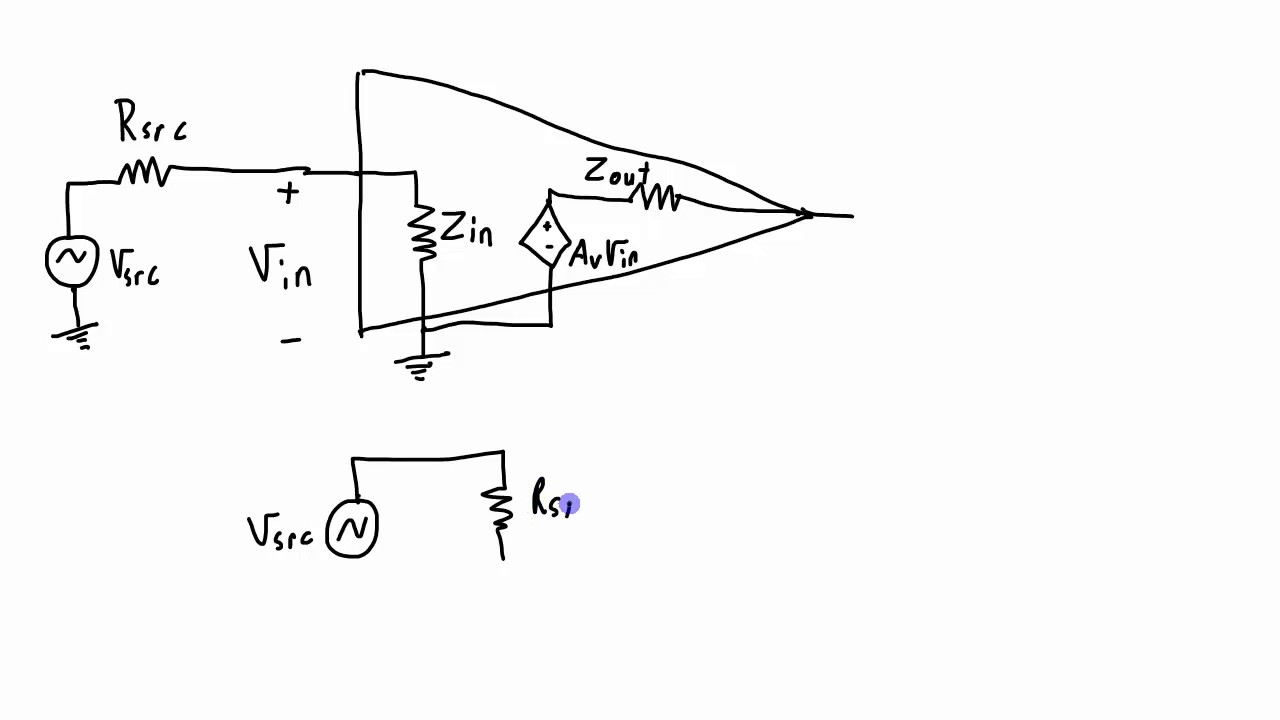The set of videos below describes three of the most important concepts of voltage amplifiers, that is the voltage gain, the input impedance, and the output impedance.  (There are of course many other amplifier characteristics, such as bandwidth, common-mode rejection, power supply rejection, noise characteristics)

# Voltage Gain

Voltage gain is the multiplying factor an amplifier applies to the signal that is input to it. The voltage at the input gets amplified (multiplied) by the gain to give an output with a new (generally higher) amplitude. The amplification factor or gain can be stated strictly as a number (which is the factor by which the output is bigger than the input) or as a decibel. The video below goes in to all of these details